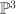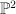## B. Siebert: “A Tropical View on Landau-Ginzburg Models”

Here are my notes from Siebert’s talk at the Extremal Laurent Polynomials workshop at Imperial:

Siebert_London_Sep_2010

Summary:

• An overview of toric degenerations and the Gross–Siebert picture
• Examples: a pencil of quartics in$\PP^3$ and a pencil of elliptic curves in$\PP^2$
• the Gross–Siebert Reconstruction Theorem
• Mirror Symmetry and the discrete Legendre Transform
• Landau–Ginzburg models; the Hori–Vafa mirror
• how to extend the superpotential from the central fiber to the whole of the mirror family in such a way that the resulting superpotential is proper
• Example:$\PP^2$, flattening the boundary of the polyhedral complex
• Broken lines and scattering